# Turning Ideas Into Code: Making Roman Numerals Count

Want to turn your ideas into code? Here’s a tip. The battle is usually won before you’ve written a line of code. Our code represents the very specific set of instructions that we give to our computers, but before we tell our computer what to do, we need to know EXACTLY what problem we are solving, and how EXACTLY we are going to solve it. This is where all the real work of programming begins and we have to rely on our problem-solving methodologies.

To get us thinking about how we can talk about the inexplicable processes our brain uses to break down problems, we can take a dive into a subject that takes very little effort for many of us: counting numbers. Counting is a task that many of us complete on a daily basis, thus it should serve as a great canvas for discussing our mental process. Let’s take a close look at writing code to understand Roman numerals, and see all the work that happens before the coding begins.

What do these 5 images have in common?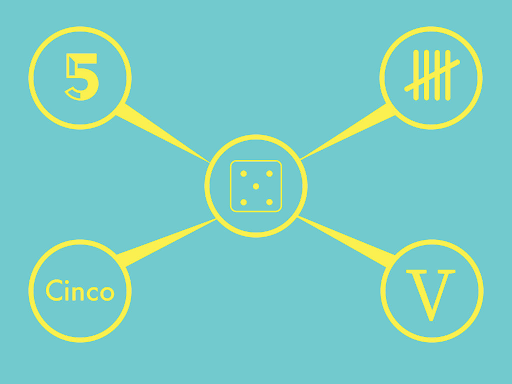You got it. They all represent the number 5.

It’s true, but what is our brain actually doing there?

One thing our minds do is convert symbols into ideas. We use these various symbols to represent the idea of the number five. The word “five” represents the number five in English. The character “5” represents the number 5 in the Arabic numeral system. Roman numerals use the symbol “V” to represent 5. There are lots of ways to represent any given number. We’ll be focusing on how numbers are represented as Roman and Arabic numerals. Specifically, we will tackle the challenge of writing code to produce Roman numerals, something a computer doesn’t do by default.

Let’s start by taking a look at how Roman numerals work. Roman numerals represent values with specific arrangements of symbols.

I = 1 V = 5 MCMLXXXIV = 1984

Roman numerals have 7 symbols that each represent a value.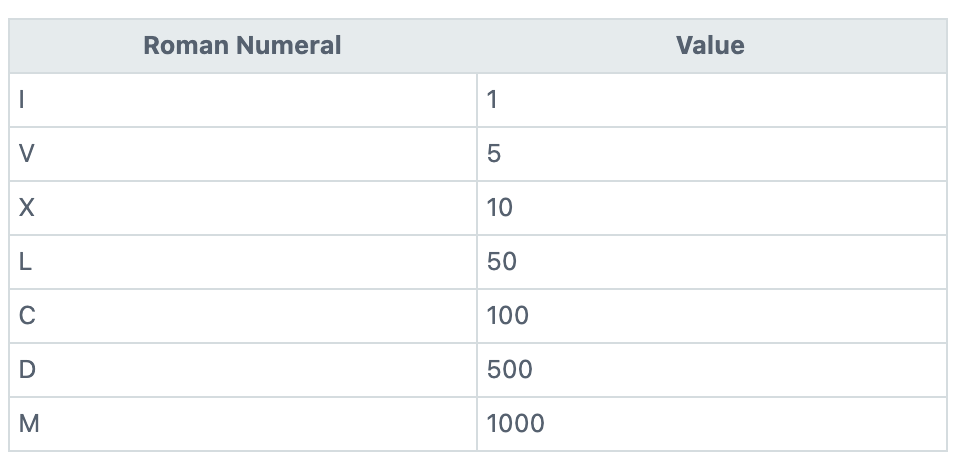Tip: If you’re like me you might forget a symbol now and then, for me it’s L and D. This silly sentence might help:

I Value Xylophones Like Cows Dig Milk

If you aren’t already familiar, check out these other equivalent representations of value: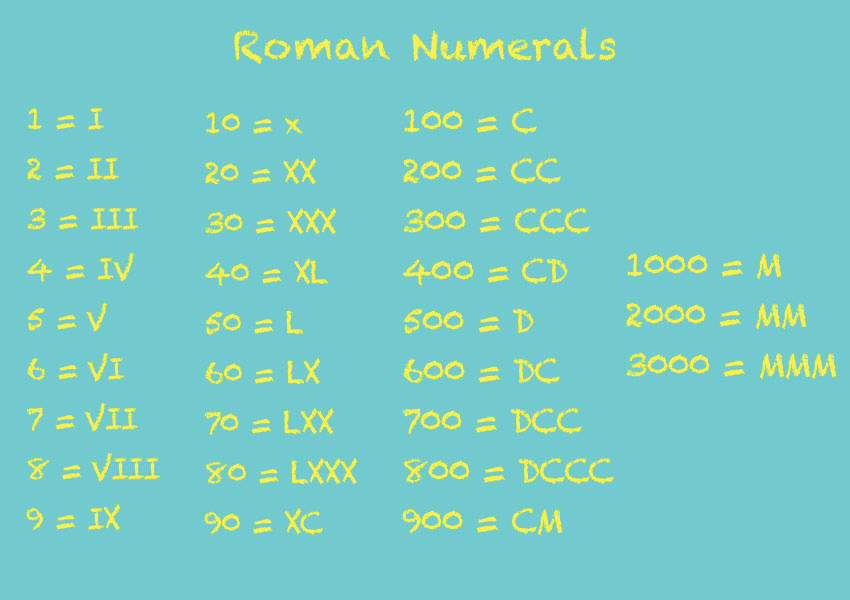Usually, each value is added to the overall total.

E.g. MMXXI equals 2021 because 1000 + 1000 + 10 + 10 + 1 = 2021In the simplest cases that’s all we need to do - just add up all the values and we’re done. However, Roman numerals also have times where a value should be subtracted instead of added.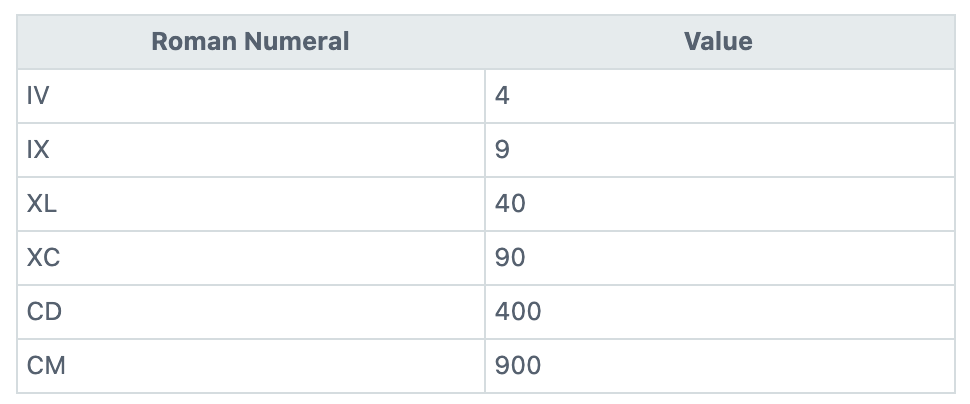See if you can spot the pattern. In these cases, the initial value is meant to be subtracted rather than added. Another way of thinking of it is that the “C” in “CM” represents -100. The general rule is that when a lesser value precedes a larger value then it should be subtracted rather than added. E.g. IX = 9 because -1 + 10 = 9So we will need to convert individual symbols into values BUT ALSO notice the order of the values to determine if we should subtract or add.

TIP: you may remember from school that “subtracting” can also be thought of as “adding the negative.” This will come in handy when we turn our ideas into code.

Jargon note: Those two styles are known as the “additive” and “subtractive” notations. Note: We are using the “standard form” of Roman numerals. This Wikipedia entry describes this (and a lot more).

The additive and subtractive notations can be used together.

Before reading further, let’s do a little exercise to make sure you have a good grasp of these concepts.

1. What does MCMLXXIV equal?
2. Can you explain exactly why?

Here is that number again broken down by value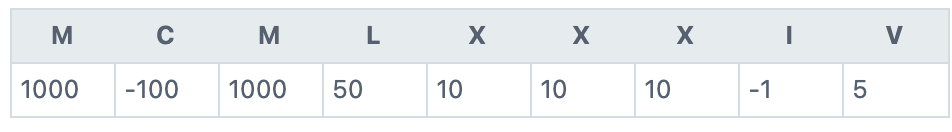So, what number did you get?

## Walk Through

To convert this process into code, we need to understand each step we take when generating a Roman numeral. Let’s do a deep-dive “walk through” of the Roman numeral MCM. We’ll start at the left and examine pairs of values along the way. We’ll refer to each item in the pair as “left” and “right”.

• To begin with, our total is 0, we’ll add to it as we go
• The left character in the pair is M
• Left value in pair equals 1000
• The right character in the pair is C
• Right value in pair equals 100
• The right value (100) is NOT less than the left value (1000)
• Add left value (1000) to our total
• Total now equals 1000 (0 + 1000)
• Move right ONE character
• The pair is now C and M
• The left character in the pair is C
• The left value in the pair is 100
• The right character in the pair is M
• Right value equals 1000
• The left value (100) IS INDEED LESS than the right value (1000) so we want to subtract the left value
• Subtract the left value (100) from our overall total
• The total is now 900 (1000 - 100)
• We won’t move ahead one character this time because we are at the last pair
• But we want to make sure to add the very last value
• The last character is M so the last value is 1000
• Add the last pair’s value to the overall total
• The overall total is now 1900 (900 + 1000)
• MCM does indeed equal 1900
• Done!

## Time for code yet?

It’s not time to write code yet, but it is time for some well defined “computational thinking,” which is well-described by Dr. Wing:

Computational thinking is the thought processes involved in formulating a problem and expressing its solution(s) in such a way that a computer – human or machine – can effectively carry out. - Jeanette M. Wing Computational Thinking Benefits Society

No code yet?? It’s ok, that’s good. There is little use telling the computer how to help solve a problem until we know how to solve it ourselves. So before we move on to writing code let’s see if we can break down all the steps involved and come up with a “recipe” to solve the problem.

The word for this “recipe” in computer science is Algorithm. The algorithm is closely related to the walk-through we just did. The previous walk-through was taking notes for the steps taken to solve the problem. The algorithm is the set of instructions given to the computer (machine or otherwise) to solve the problem.

### ALGORITHM

• Start at the first character and repeat the steps until you reach the second to last character, one character at a time.
• The current character is `left` in the pair
• The following character is `right` in the pair
• Convert `left` and `right` characters to numbers
• If the left value is less than the right value then subtract the left value
• Otherwise, add the left value to the total
• Repeat as needed
• Convert the last character to a number
• Return the total

### Another Quick Stroll

Let’s confirm our Algorithm is correct by doing another walkthrough.

Let’s try CXLII, which should equal 142 if the algorithm is correct.

• Total = 0
• repeat from first character to second-to-last, one at a time
• The first pair = CXL
• The left number = 100
• The right number = 10
• The left is NOT less than the right
• The total = 0 + 100
• The second pair = XL
• The left number = 10
• The right number = 50
• The left number IS less than the right number so subtract the left value
• The total = 100 + -10 (90)
• The third pair = LI
• The left number = 50
• The right number = 1
• The left number is NOT less than the right number
• The total = 90 + 50 (140)
• The fourth pair = II
• The left number = 1
• The right number = 1
• The left number is NOT less than the right number
• The total = 140 + 1 (141)
• Done repeating
• Last character = I
• Last value = 1
• Total = 141 + 1 (142)
• Done!

## Code

Now that we’ve broken down our solution step by step it’s finally time to get the computer involved. If you’ve really “decomposed” the problem then often the code can flow nicely from the algorithm.

Here is one possible solution written in the Python programming language. Lots of developers like Python because it reads almost like English. If you like the look of Python you may be interested in the Advanced Software Development in Python course here at Code Fellows.

See if you can track how each line of code relates back to the algorithm.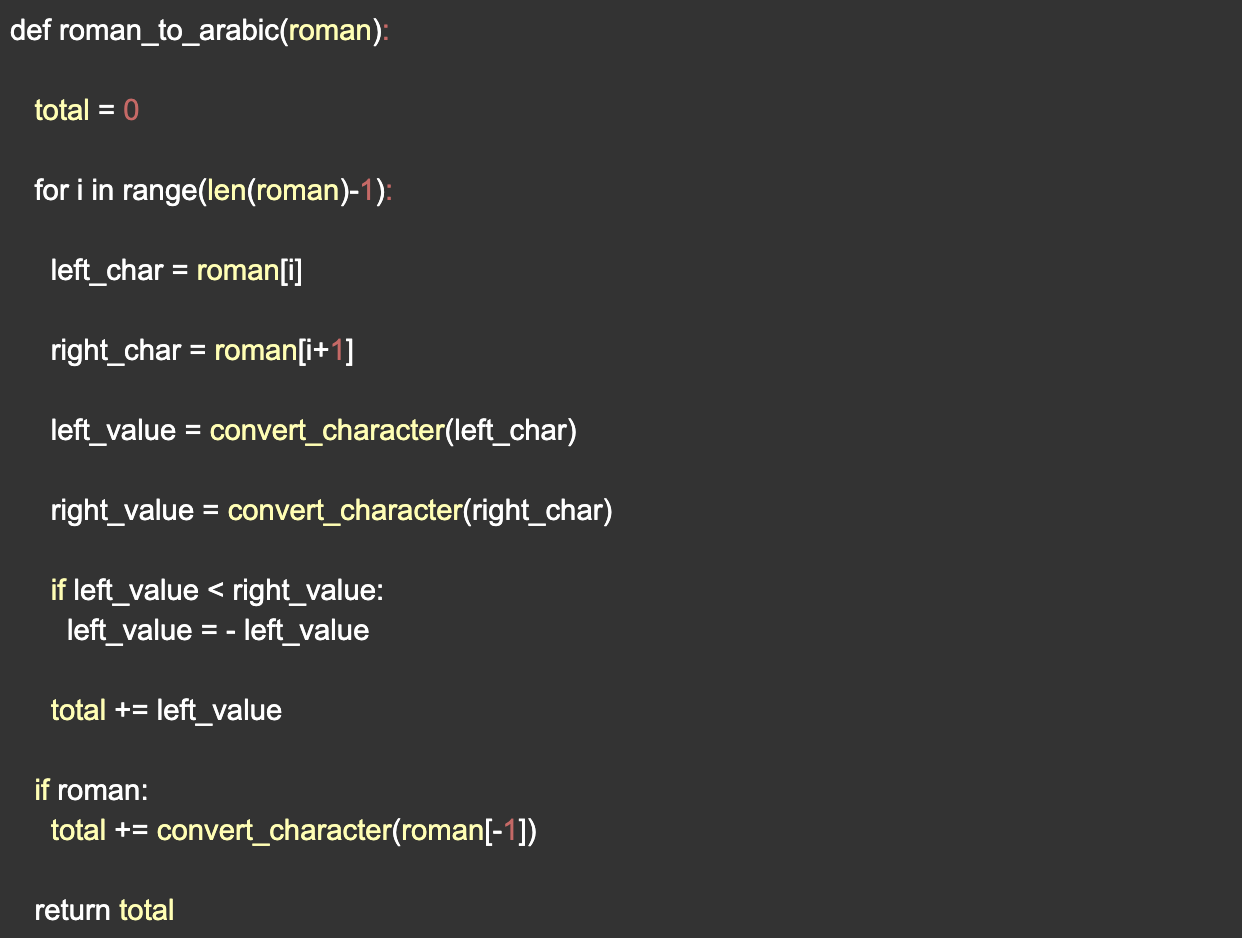The code reads better if the job of converting a Roman numeral character is moved to a separate function.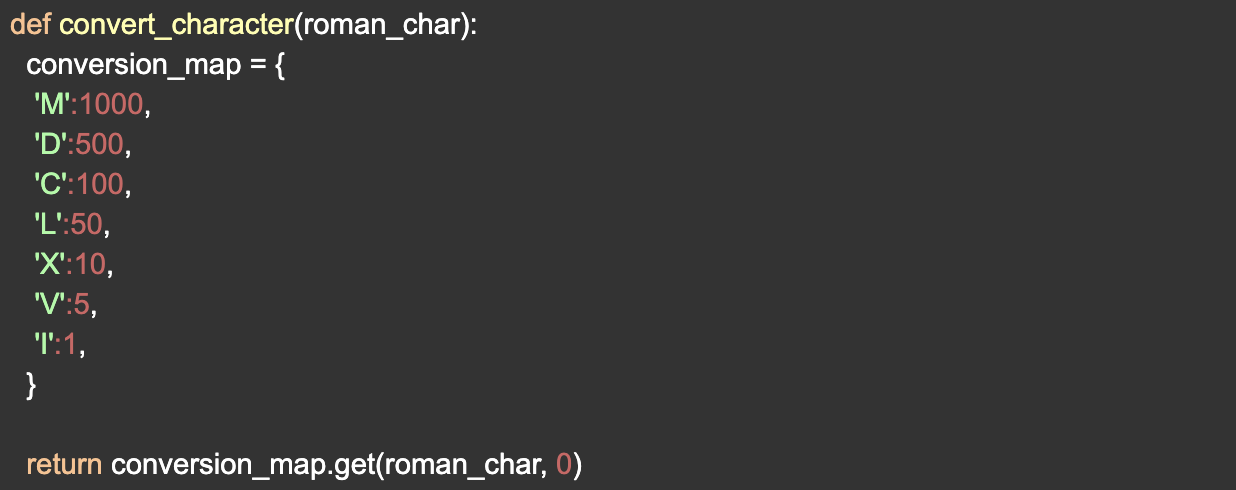## Summary

There’s a saying, “You can’t expect the computer to know what you want if you can’t say what you want.” In other words, in order to write good code, we need to have a good idea of what the code needs to do. Computational thinking helps us start with something general, and work our way toward identifying specific decision points before we tell the computer what decisions to make.

Get it straight in your head, then tell the computer what steps to take. This is how you turn ideas into code!